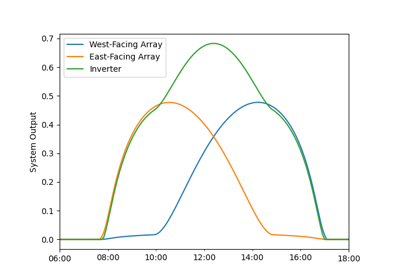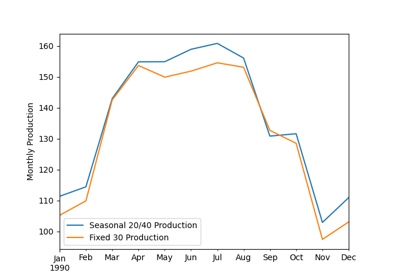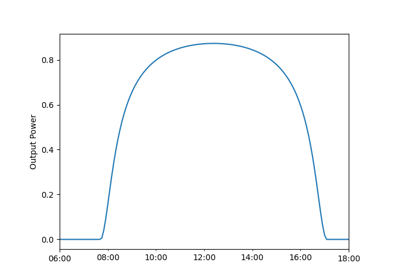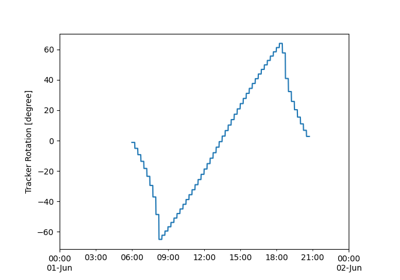# pvlib.modelchain.ModelChain.run_model#

ModelChain.run_model(weather)[source]#

Run the model chain starting with broadband global, diffuse and/or direct irradiance.

Parameters

weather (DataFrame, or tuple or list of DataFrame) –

Irradiance column names must include `'dni'`, `'ghi'`, and `'dhi'`. If optional columns `'temp_air'` and `'wind_speed'` are not provided, air temperature of 20 C and wind speed of 0 m/s are added to the DataFrame. If optional column `'cell_temperature'` is provided, these values are used instead of temperature_model. If optional column module_temperature is provided, temperature_model must be `'sapm'`.

If list or tuple, must be of the same length and order as the Arrays of the ModelChain’s PVSystem.

Returns

self

Raises
• ValueError – If the number of DataFrames in data is different than the number of Arrays in the PVSystem.

• ValueError – If the DataFrames in data have different indexes.

Notes

Assigns attributes to `results`: `times`, `weather`, `solar_position`, `airmass`, `total_irrad`, `aoi`, `aoi_modifier`, `spectral_modifier`, and `effective_irradiance`, `cell_temperature`, `dc`, `ac`, `losses`, `diode_params` (if dc_model is a single diode model).

## Examples using `pvlib.modelchain.ModelChain.run_model`#